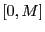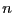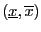Next: Mathematical background Up: Analyzing trigonometric equations Previous: Introduction   Contents

# Number of roots of trigonometric equation

The purpose of this section is to present an algorithm which enable to determine how many roots has an equationin the unknownof the form:(4.1)

withinandin, bothbeing integers, andin.

Subsections

Jean-Pierre Merlet 2012-12-20